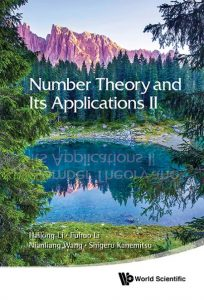Book Reviews bring interesting mathematical sciences and education publications drawn from across the entire spectrum of mathematics to the attention of the CMS readership. Comments, suggestions, and submissions are welcome.

Karl Dilcher, Dalhousie University (notes-reviews@cms.math.ca)Number Theory and its Applications
by Fuhuo Li, Nianliang Wang, Shigeru Kanemitsu
World Scientific, 2013
ISBN 978-981-4425-63-6

Number Theory and its Applications II
by Hailong Li, Fuhuo Li, Nianliang Wang, Shigeru Kanemitsu
World Scientific, 2018
ISBN 978-981-3231-59-7

Reviewed by Keith Johnson, Dalhousie University

In the preface of the first of these two books about number theory the authors describe their intent as “starting from the very basics and warp into the space of of new interest; from the ground state to the excited state”. By the latter they mean certain parts of analytic number theory. Each book is organized into six chapters, with two chapters of introductory material followed by four more or less independent advanced topics. An unusual feature of the books is that the second, published 5 years after the first, not only covers much of the same material as the first, but about one quarter of it is selected sections reprinted verbatim from the first book. The result is that the second book is more than a second edition but less than a second volume.

The first book starts with a chapter of elementary material on algebra and algebraic number theory, but with examples and exercises (most with included solutions) looking forward to analytic number theory. Emphasis is placed on the “gcd principle” that if a family of statements P(n) is such that P(n) and P(m) implies P(gcd(n,m)) then the minimal f for which P(f) has the property that P(n) implies f divides n. this is used as the basis for a discussion of arithmetic functions, and so as a means of introducing Dirichlet characters at an early stage.  The second chapter, on algebraic number theory, states the basics of Galois theory, describes the structure of modules over a Dedekind domain, and then concentrates on algebraic number fields. The basic facts about trace and norm and about valuations are given, and then three specific examples are developed: the quadratic field with golden ratio unit, cyclotomic fields (which leads to the statement of Dirichlet’s prime number theorem), and fields with the dihedral group as Galois group.

Following these two chapters of “basics”, the book has four chapters of “interesting” material. First is a chapter on arithmetic functions with material on asymptotic formulas, on generating functions, and an application to sequences in Hilbert spaces. Next is achapter on quadratic reciprocity including a discussion of Hecke’s theorem on the quadratic reciprocity law in algebraic number fields, although without a complete proof. This is followed by a chapter dedicated to Dirichlet L-functions, mostly concentrating on those with primitive characters. There is another proof of quadratic reciprocity using the theta transformation formula, material on Lambert series and character sums, and a discussion of the discrete mean square of special values of L-functions. The final chapter describes some connection between analytic number theory and control theory. The basics of control theory are sketched and then the application of analytic methods to find controllers satisfying specified stability and bound conditions are described.

As mentioned earlier, the second book follows the same six chapter format with two basic chapters followed by four interesting ones. Chapter one adds material about number fields by emphasising the use of techniques from linear algebra. Chapter two emphasises the use of results from group theory and develops the Galois theory of number fields more completely. The examples of number fields from the first book are reprinted here and additional material such as L-functions associated to elliptic curves is added. Chapter three reprints four sections of material about arithmetic functions and then gives a variety of results about average values of arithmetic functions and error estimates for these. Chapter four develops further results about Lambert series, continuing from chapter three of the first book, and looks at various results of Riemann including his example of a continuous non-differentiable function. Chapter five continues the study of the connections between number theory and Hilbert spaces, reprinting the corresponding section from the first book and then developing and proving Kuznetsov’s trace formula. Chapter six continues the number theoretic study of control theory, reprinting several sections from the first book and adding several illustrative examples from electrical engineering.

These books present a wide range of interesting number theoretic topic and the reader most likely to enjoy them and benefit from their study would be someone already well versed in analytic number theory. I don’t think either would be successful as a textbook, in spite of the excellent solved exercises scattered throughout, because of the diversity of topics and the lack of a central organizing theme. Instead its most likely user will be an instructor using it as a source of ideas to supplement some other, more coherent, textbook.